Courses

# Test: Continuity Equation

## 15 Questions MCQ Test Fluid Mechanics | Test: Continuity Equation

Description
This mock test of Test: Continuity Equation for Mechanical Engineering helps you for every Mechanical Engineering entrance exam. This contains 15 Multiple Choice Questions for Mechanical Engineering Test: Continuity Equation (mcq) to study with solutions a complete question bank. The solved questions answers in this Test: Continuity Equation quiz give you a good mix of easy questions and tough questions. Mechanical Engineering students definitely take this Test: Continuity Equation exercise for a better result in the exam. You can find other Test: Continuity Equation extra questions, long questions & short questions for Mechanical Engineering on EduRev as well by searching above.
QUESTION: 1

### If a liquid enters a pipe of diameter d with a velocity v, what will it’s velocity at the exit if the diameter reduces to 0.5d?

Solution:

Explanation: According to the Continuity Equation,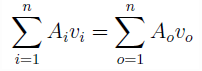where a represents flow area, v represents flow velocity, i is for inlet conditions and o is for outlet conditions.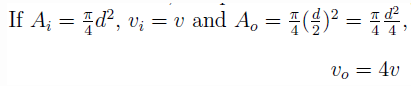QUESTION: 2

### The continuity equation is based on the principle of

Solution:

Explanation: According to the Continuity Equation, if no fluid is added or removed from the pipe in any length then the mass passing across different sections shall be the same. This is in accordance with the principle of conservation of mass which states that matter can neither be created nor be destroyed.

QUESTION: 3

### wo pipes of diameters d1 and d2 converge to form a pipe of diameter d. If the liquid flows with a velocity of v1 and v2 in the two pipes, what will be the flow velocity in the third pipe?

Solution:

Explanation: According to the Continuity Equation,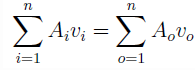where a represents flow area, v represents flow velocity, i is for inlet conditions and o is for outlet conditions. Thus,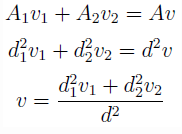QUESTION: 4

In a two dimensional flow, the component of the velocity along the X-axis and the Y-axis are u = ax2 + bxy + cy2 and v = cxy. What should be the condition for the flow field to be continuous?

Solution:
QUESTION: 5

Two pipes, each of diameter d, converge to form a pipe of diameter D. What should be the relation between d and D such that the flow velocity in the third pipe becomes double of that in each of the two pipes?

Solution:

Explanation: According to the Continuity Equation,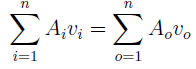where a represents flow area, v represents flow velocity, i is for inlet conditions and o is for outlet conditions. Thus,
A1v1 + A2v2 = Av
d2v + d2v = D2v
D = d.

QUESTION: 6

Two pipes, each of diameter d, converge to form a pipe of diameter D. What should be the relation between d and D such that the flow velocity in the third pipe becomes half of that in each of the two pipes?

Solution:

Explanation: According to the Continuity Equation,where a represents flow area, v represents flow velocity, i is for inlet conditions and o is for outlet conditions. Thus,
A1v1 + A2v2 = Av
d2v + d2v = D2(v/2)
d = D ⁄ 2.

QUESTION: 7

In a two dimensional flow, the component of the velocity along the X-axis is u = ax2 + bxy + cy2.
If v = 0 at y = 0, what will be the velocity component in the Y-direction?

Solution:

Explanation: According to the condition for continuity,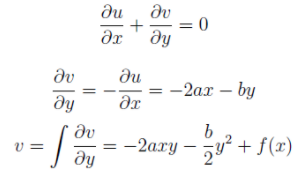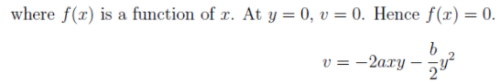QUESTION: 8

In a two dimensional flow, the component of the velocity along the X-axis and the Y-axis are u = ax2 + bxy + cy2 and v = cxy. What should be the condition for the flow field to be continuous?

Solution:

Explanation: According to the condition for continuity,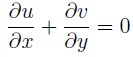2ax + cx = 0
2a + c = 0.

QUESTION: 9

In a two dimensional flow, the component of the velocity along the X-axis and the Y-axis are u = axy and v = bx2 + cy2. What should be the condition for the flow field to be continuous?

Solution:

Explanation: The condition for the flow field to be continuous is: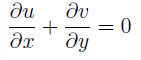ay + 2cy = 0
a + 2c = 0.

QUESTION: 10

In a two dimensional flow, the component of the velocity along the X-axis and the Y-axis are u = ax2 + bxy and v = cxy +dy2. What should be the condition for the flow field to be continuous?

Solution:

Explanation: The condition for the flow field to be continuous is:2ax + cx + by + 2dy = 0
(2a + c)x + (b + 2d)y = 0.

QUESTION: 11

In a two dimensional flow, the component of the velocity along the X-axis and the Y-axis are u = ax2 + bxy and v = bxy + ay2. The condition for the flow field to be continuous is

Solution:

Explanation: The condition for the flow field to be continuous is:2ax + by + 2ay + bx = 0
x + y = 0
Hence, the condition for the flow field to be continuous is independent of the constants (a; b) and dependent only on the variables (x; y).

QUESTION: 12

In two dimensional flow the components of velocity are given by u = ax; v = by. The stream lines will be

Solution:
QUESTION: 13

In a two dimensional flow, the component of the velocity along the X-axis and the Y-axis are u = ay2 + bxy and v = ax2 + bxy. The flow will be continuous if

Solution:

Explanation: The condition for the flow field to be continuous is:by + bx = 0
x + y = 0.

QUESTION: 14

In a two dimensional flow, the component of the velocity along the X-axis and the Y-axis are u = ax2 + bxy and v = bxy + ay2. The condition for the flow field to be continuous is

Solution:

Explanation: The condition for the flow field to be continuous is:2ax + by + 2ay + bx = 0
x + y = 0
Hence, the condition for the flow field to be continuous is independent of a, b and c.

QUESTION: 15

In a water supply system, water flows in from pipes 1 and 2 and goes out from pipes 3 and 4 as shown. If all the pipes have the same diameter, which of the following must be correct?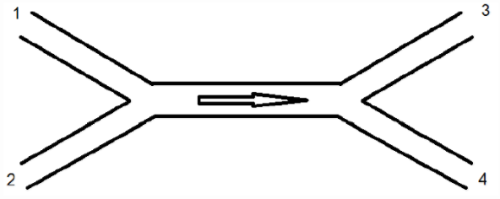Solution:

Explanation: According to the Continuity Equation,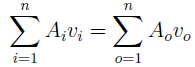where a represents flow area, v represents flow velocity, i is for inlet conditions and o is for outlet conditions.
A1v1 + A2v2 = A3v3 + A4v4
Since d1 = d2 = d3 = d4, v1 + v2 = v3 + v4.